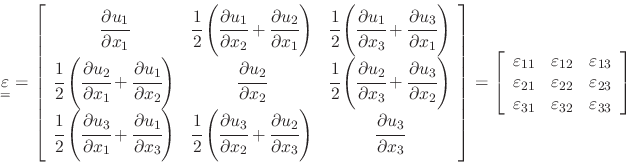# 3.2 Kinematic equations: displacements and strains

Applications of stresses result in solid deformation and displacements. Figure 3.7 shows an example of a solid body and the corresponding displacement vector field (traces the displacements from the original to the deformed state). In this particular case, the solid is anchored at the bottom and deforms due to the application of a force (from left to right) on the top. Hence, displacements at the bottom are zero and displacements on the top are the maximum.Yet, absolute displacements are not enough to determine stresses. A solid may translate or rotate in space without development of any internal stresses required to equilibrate external actions (imagine a cookie “floating” in zero gravity within the International Space Station https://www.youtube.com/watch?v=q5uV4fTV0Zo).

Let's look at Figure 3.8 in order to relate displacements to strains:

• (LEFT) A solid is stretched on its face 1 (perpendicular to) in direction 1 only. This type of deformation produces a change of volume of the solid and therefore contributes to volumetric strain. The resulting deformation or strain (change of length divided original length) is(3.4)

• (CENTER) A solid is stretched on its face 2 in direction 2 only. This type of deformation also contributes to volumetric strain. The resulting strain is(3.5)

• (RIGHT) The solid is now distorted. Notice that the faces do not make a right angle anymore. The change of volume is negligible for small deformations. The resulting distortion or shear strain is proportional to the change in angle between faces 1 and 2. Hence the change of angle is. The shear strain is 1/2 of the total change of the angle and therefore (for small changes)(3.6)

The average in the equation ensures capturing shear distortion rather than rotation.Strains do not quantify the absolute value of displacements, but its variation in space (derivative with respect to). All other strains are found with similar equations in the 3D case. Similarly to the stress tensor, strains can be organized in a tensor where elements in the diagonal contribute to volumetric strain, and off-diagonal elements are shear strains.(3.7)

The summation of all diagonal terms yields the volumetric strain(3.8)

EXAMPLE 3.2: Demonstrate the equality in Eq. 3.8 from simple geometrical concepts. Hint: the initial volume of the solid is.

SOLUTION
The definition of volumetric strain is the ratio between the change of volumeand the initial volume:For an elementary cubic volume with initial volumeand volume after deformation, the equation above resultsLet us discard all the products containingand alsobecause they are much smaller than the other terms including just one(), then